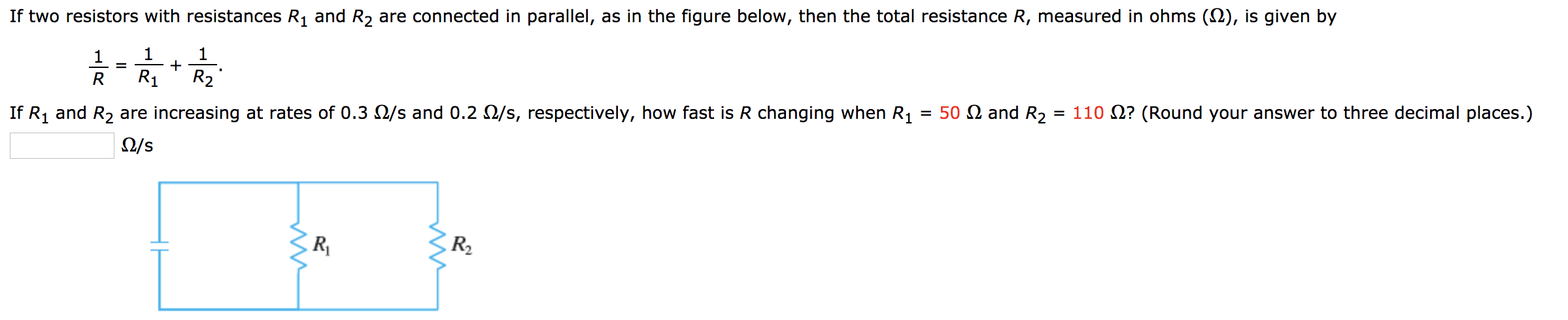# and R2 are connected in parallel, as in the figure below, then the total resistance R, measured in ohms (2), is given by If two resistors with resistances 1 1 1 R1 R2 R = 50 2 and R2 If R1 and R2 are increasing at rates of 0.3 2/s and 0.2 O/s, respectively, how fast is R changing when R1 = 110 ? (Round your answer to three decimal places.) 2/s RV

Questionhelp_outlineImage Transcriptioncloseand R2 are connected in parallel, as in the figure below, then the total resistance R, measured in ohms (2), is given by If two resistors with resistances 1 1 1 R1 R2 R = 50 2 and R2 If R1 and R2 are increasing at rates of 0.3 2/s and 0.2 O/s, respectively, how fast is R changing when R1 = 110 ? (Round your answer to three decimal places.) 2/s RV fullscreen